# Work, Energy & Momentum Flashcards Preview

## AP Physics > Work, Energy & Momentum > Flashcards

Flashcards in Work, Energy & Momentum Deck (86):
1

2

3

4

5

6

7

8

9

10

11

## A force F1 is exerted a distance d1 from the fulcrum of a lever. What does the force F2 at d2 from the other end equal?12

13

14

15

16

17

18

19

20

21

22

23

24

25

26

27

28

29

30

31

32

33

34

35

36

37

38

39

40

41

42

43

44

45

46

47

48

49

50

51

52

53

54

55

56

57

## translation (as it applies to physics)

### Translation is the net movement or change in location of an object. This may be thought of as a non-zero displacement in a direction.  Ex: a hockey puck may be said to have experienced translation when it began at one end of an ice rink and was later at the other end.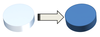58

59

60

## fulcrum and lever arm

### The fulcrum is the point of an object that remains fixed during rotation. The lever arm is the distance from the fulcrum where a force is applied, to create rotation around the fulcrum.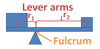61

## torque

### Torque is the ability of a force to create a change in the angular orientation of an object, by rotation about a fulcrum point. Torque can also be thought of as the component of work perpendicular to a lever arm.  The SI unit of torque is the N-m.62

63

64

## Describe the convention of rotation that is exibited from a positive torque.

### Counter-clockwise rotation is positive torque. The maximum positive (counter-clockwise) torque that a force can deliver is at 90 degrees to the lever arm.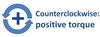65

## Describe the convention of rotation that is exibited from a negative torque.

### Clockwise rotation is negative torque. The maximum negative (clockwise) torque that a force can deliver is at 90 degrees to the lever arm.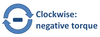66

67

68

## A child of mass 40kg sits 1.5m from the pivot of a see-saw. To balance exactly, where on the opposite end should her 30kg little sister sit? (assume the seesaw is massless)### She should sit 2m from the pivot point.  Since all forces due to gravity are perpendicular to the see-saw, torque from the 40kg child will be: (mg)r = 40(10)(1.5) = 600 Nm. To balance, the 30kg child needs to supply 600 Nm of torque, which requires a distance of 2m. On the AP B exam, torque questions that require calculation always ask for the state of rotational equilibrium (on the AP C exam, further calculations are possible).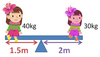69

## What visual tool can be used to account for all forces acting on an object?

### A free-body diagram.  Free-body diagrams represent force vectors with arrows pointing in the corresponding direction. Larger forces are represented by longer arrows.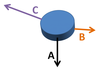70

## Describe/draw the free-body diagram for a box with mass m, accelerating towards the earth in free fall.

### A force due to gravity, pointing directly downwards with magnitude mg. A force due to air resistance, pointing directly upwards with magnitude kv2 (the negative sign is only necessary for calculations). Note: since the mass is accelerating downwards, the arrow for gravity is represented larger than for air resistance.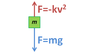71

## Describe/draw the free body diagram for the stationary block of mass m on the slope below, providing specific values where possible.### A force due to gravity pointing directly downwards, with magnitude mg.  A normal force pointing perpendicular to the slope, with magnitude mg cos(θ). A static friction force pointing parallel to the slope, opposing gravity, with a magnitude of mg sin(θ). Note: the length of the arrows should be proportional to the magnitude of each force.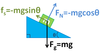72

73

74

75

76

77

## Explain with an example whether it is possible for objects in an isolated system to collide and stop.

### It is possible. Consider two cars of equal weight and equal-but-opposite velocity. Conceptually, this means that the system's initial momentum is zero, since each car's momentum is equal and opposite the other's. If they collide and stick (totally inelastic collision), then the final object's momentum must be zero - hence, both cars come to rest together.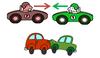78

79

## Two ideal hockey pucks of equal mass, one traveling to the right and the other to the left, collide in space. Describe the motion of the pucks after collision, assuming they began with equal speed.### The pucks will bounce off of each other in a perfectly elastic collision.  The puck originally traveling left will now go back right, and the puck originally traveling right will now go back left. Their speeds and masses remain constant, but the direction (sign) of velocity and momentum will now be opposite.80

81

## Two cars of equal mass, one traveling to the right and the other to the left, collide and deform in space. Describe the motion of the cars after collision, assuming they began with equal speed and are not stuck together afterwards.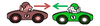### The cars will bounce off of each other in an inelastic collision.  The cars will lose kinetic energy due to the collision. Their speeds will decrease and masses may change if one car loses mass to the other. The direction (sign) of velocity and momentum will now be opposite, but total momentum remains conserved.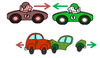82

83

## Two soft clay balls of equal mass, one traveling to the right and the other to the left, collide and stick in space. Describe the motion of the balls after collision, assuming they began with equal speed.### The balls will stick together and stop, in a totally inelastic collision.  The balls will lose kinetic energy due to the collision. Their speeds will decrease to zero and masses combine together. The velocity will now be zero, but total momentum remains conserved (it began as zero).84

## Two balls of equal mass collide in space. Knowing nothing else, what can be said about this system after collision? What if the collision was elastic vs. totally inelastic?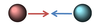85

86